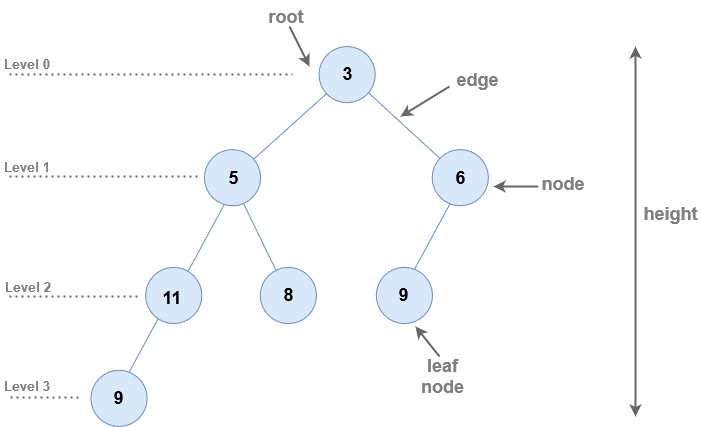# Array Implementation of Binary Tree

## Array Implementation Of Binary Tree

There are multiple ways of implementing a binary tree. In this article, we will be discussing about the array implementation of binary trees

For example, if we want to implement a binary tree given below as an array :Since, this binary tree contains 3 levels, to implement this tree as an array, we need
`1 + 2^0 + 2^1 + 2^2 + 2^3 = 16` nodes. After that, we will store the level order traversal of the tree into the array.
So the array would look like this :
`[-1, 3, 5, 6, 11, 8, 9, -1, 9, -1, -1, -1, -1, -1, -1, -1 ]`
`-1` in the above array means that the corresponding position is blank.
Note that the `0th` position in the above array is intentionally left blank.

### Parent

Using the above array implementation of binary trees, we can use the following function to find parent of any node situated at position `i`.

``````Parent(i) {
return i/2
}
``````

For example, the node with value `8`, is situated at position `5` within the array. Therefore, according to the `Parent` function above, it’s parent will be located at index `5/2 = 2`. The node at position `2` is `5`, which is indeed the parent of node `8` as you can check from the diagram above.

### Left Child

Using the array implementation of binary trees, we can use the following function to find left child of any node situated at position `i`

``````LeftChild(i) {
return 2*i
}
``````

For example, the node with value `5` is situated at position `2`. Therefore, according to the above function, it’s left child will be located at position `2*2 = 4`. The element at position `4` is `11`, which is indeed the left child of `5` as can be seen from the diagram above.

### Right Child

Using the array implementation of binary trees, we can use the following function to find the right child of any node situated at position `i`.

``````RightChild(i) {
return 2*i + 1
}
``````

For example, the node with value `5` is situated at position `2` in the array implementation of binary tree. Therefore, according to the above function, it’s right child will be located at position `2*2 + 1 = 5`. The element at position `5` is `8`, which is indeed the right child of `5` as can be seen from the diagram above.

Scroll to Top
[gravityforms id="5" description="false" titla="false" ajax="true"]